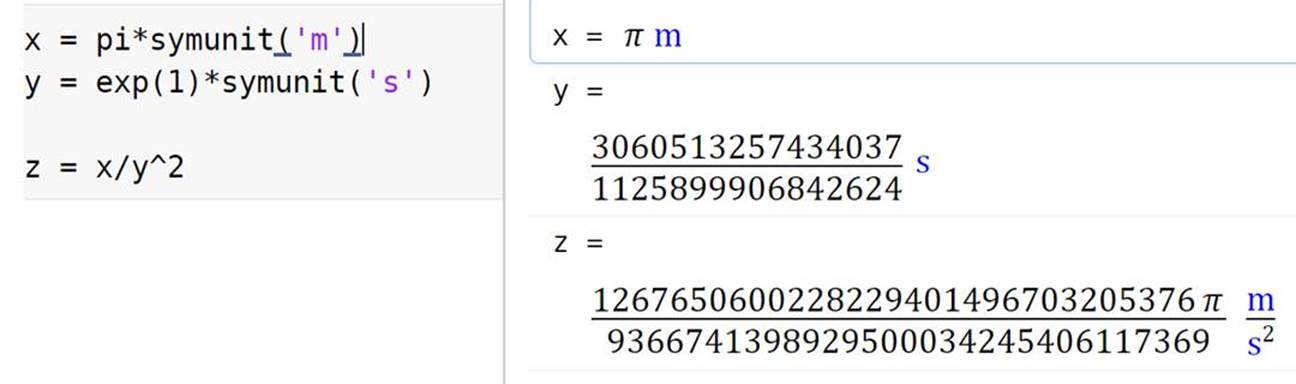# Physical Units in MATLAB

### Contents

#### Physical Units in MATLAB

Greg’s pick this week is Physical Units Toolbox by Sky Sartorius.

Wouldn’t it be nice to have units to go with those numbers you compute in MATLAB?

% Velocity in kilometers per hour
v = 12.3 * u.kph
% Time in seconds
t = 3.7 * u.second
% Distance travelled
d = v * t
% Convert distance to feet
u2num(d, u.foot)
% Another method to convert distance to feet
d/u.ft

v =

3.4167

m
-
s

t =

3.7000

s

d =

12.6417

m

ans =

41.4753

ans =

41.4753



I’ve always wanted this functionality in both MATLAB and Simulink. When I was a student in University, MATLAB was my favorite programming tool, but I didn’t use it much for engineering homework, as there were other tools that had nice worksheets and included units with unit conversions and computations.

Even as an employee of MathWorks I’ve found myself using other math software to assist with computations that required careful tracking and conversion of units.

#### Why did I pick this entry?

The two key elements for me are it is:

• Easy to use
• Well documented

The inclusion of units by just simply multiplying by the desired unit is a well thought out mechanism. Also, there are of course functions and methods to extract values of a specific unit type.

And there is unit consistency checking, making sure that you don’t attempt to do things like convert a distance to a temperature.

try
u2num(d, u.kelvin)
catch Me
disp(Me.message);
end

Incompatible input dimensions


The attention to detail and planning the functionality provides for easy use of this tool. For example, Sky included functions for unit conversion for standard MATLAB variables, not just the unit class. This means you don’t have to think about whether or not a particular variable is of the DimVar class or not.

a = pi;
u2num(a)

ans =

3.1416



#### Extending capabilities of the class

The organization and documentation of the code makes it pretty easy to add additional functions that enable conversions. For example, many times I may want to include the result of a particular computation as a variable in Simulink. With the addition of units in Simulink (R2016a) there can be consistency between the environments.

g = 9.81 *u.m/u.s^2
% Create Simulink.Parameter object with the same value and units as "g"
gParam = u2param(g)

g =

9.8100

m
---
s^2

gParam =

Parameter with properties:

Value: 9.8100
Description: ''
DataType: 'auto'
Min: []
Max: []
Unit: 'm/s^2'
Complexity: 'real'
Dimensions: [1 1]



Now the units engine in Simulink will be able to help with things like units consistency checking when this variable “gParam” is used in the Simulink model.

type u2param
type getUnitString

function p = u2param(v)

assert(isa(v, 'DimVar'));

s = getUnitString(v);

p.Unit = s;
end

function s = getUnitString(v)

assert(isa(v, 'DimVar'));

[num, dem] = display(v);

s = sprintf('%s/%s', num, dem);

end


#### Getting the unit text

Getting the units of a value as a string that can be interpreted by other MATLAB and Simulink tools would be helpful.

Technically the UNITSOF function returns the unit string of an object, but in a specialized format.

unitsOf(g)

[m^1][s^-2]


This can be remedied pretty easily with the following:

[numerator, denominator] = display(g);
[numerator '/' denominator]

ans =

'm/s^2'



And of course as or R2017a, you can do things like:

numerator + "/" + denominator

ans =

"m/s^2"



#### MathWorks tools that have units

There are some addons that do support physical units.

Simulink now has a notion of units on signal lines.

The Simscape products all require the use of specific units when defining properties of physical elements.

The Symbolic Math Toolbox also has some capability to apply physical units to calculations and enable symbolic manipulations as well.#### Should MATLAB have a units system built in?

Let us know here.

Published with MATLAB® R2017a

|